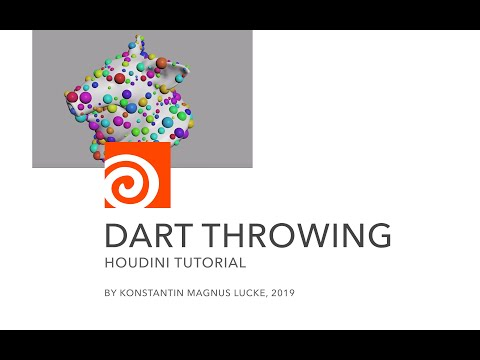# Dart Throwing Algorithm

calendar_today

06.06.2023

label

Modeling, VEX

mouse

Houdini 19.5

### 1 Description

Populating a mesh surface with non-intersecting spheres using the dart throwing algorithm inside a Houdini solver.

### 2 Code discussion

Every `@Frame` a random `uvw`-position is tested for its distance to the uv islands of the mesh with the `uvdist`-function. If `dist < 1e-5` the random UV position is either on the island or at least very close to its boundary. Then the corresponding world `pos`ition is returned by the `primuv`-function and only if no other point `len(pts) != 1` can be found `pcfind` within a randomly defined `radius` a new point is created. The new point's radius is written into the `pscale` attribute with `setpointattrib` so it can potentially be found in the next iterations.

``````vector uvw = rand(@Frame, 123);
uvw = 0.0;

int pr;
vector st;
float dist = uvdist(1, 'uv', uvw, pr, st);

if(dist < 1e-5){
vector pos = primuv(1, 'P', pr, st);
float radius = fit01( rand(@Frame, 456), 0.05, 0.1 );

if(len(pts) != 1){